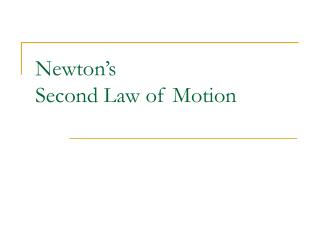DownloadDownload PresentationNewton’s Second Law of Motion

# Newton’s Second Law of Motion

Télécharger la présentation## Newton’s Second Law of Motion

- - - - - - - - - - - - - - - - - - - - - - - - - - - E N D - - - - - - - - - - - - - - - - - - - - - - - - - - -
##### Presentation Transcript

1. Newton’sSecond Law of Motion

2. Forces: Recap • Force: a push or a pull on an object; the result of an interaction between two objects • Units: newtons (N) • Types of forces: • Gravity (Fgrav) • Friction (Ffric) • Tension (Ften) • Normal Force (Fnorm) • Applied Force (Fapp) • Force is a vector quantity. Multiple forces acting on an object produce a net force.

3. Free Body Diagrams • Standard representation of therelative magnitude and directionof all forces acting upon an object. • Object represented by a box, forces by arrows • Size of the arrow reflects magnitude of force • Direction of the arrow shows force direction • Each arrow is labeled to indicate the force type • Arrows are always drawn outward from the box • Must depict all the forces which act on the object Check out www.physicsclassroom.com for a bunch of great examples!

4. Net Force • Vector sum of all forces acting on the object • Vector addition considers different directions • Same direction: add • Opposite direction: subtract • What about at right angles? • What about at obtuse or acute angles? Check out www.physicsclassroom.com for a bunch of great examples!

5. Net Force • The net force is known for each situation below. • However, the magnitudes of a few of the individual forces are not known. • Analyze each situation individually and determine the magnitude of the unknown forces.

6. Recall: Newton’s First Law of Motion • An object at rest will remain at rest andan object in motion will remain in motion at a constant velocity,unless it is acted on by unbalanced forces • This tendency increases as mass increases • So, basically…velocity doesn’t change without a net force

7. Newton’s Second Law of Motion • The acceleration of a body is directly proportional to the net force acting on the body and inversely proportional to the mass of the body. Consider this: 1 N = 1 kg·m/s2 Does that make sense?

8. Newton’s Second Law of Motion